# 一文通俗入门·脉冲神经网络(SNN)·第三代神经网络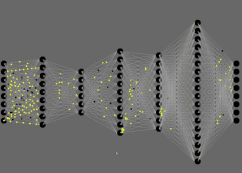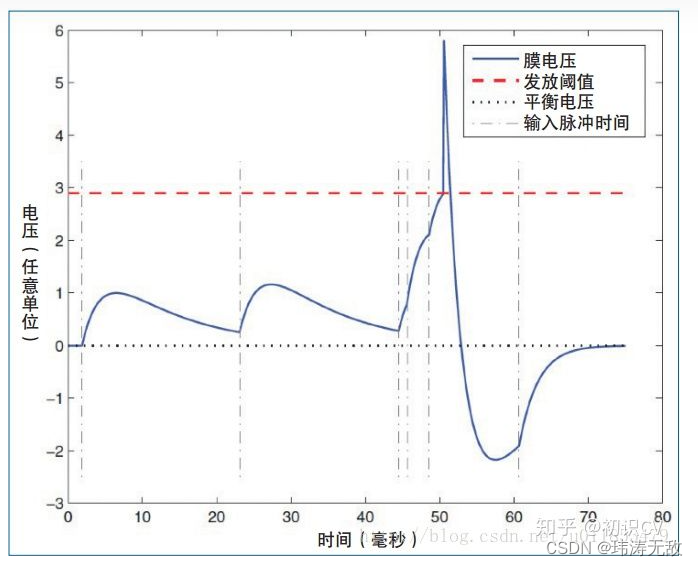# 一、脉冲神经元模型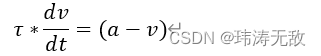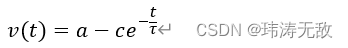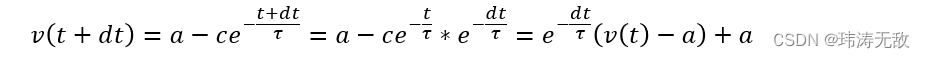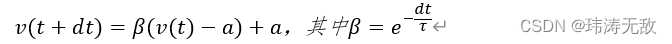``````import numpy as np
import matplotlib.pyplot as plt

fig = plt.figure(figsize=(5, 4))
ax = plt.subplot(111)

# Function that runs the simulation
# tau: time constant (in ms)
# t0, t1, t2: time of three input spikes
# w: input synapse weight
# threshold: threshold value to produce a spike
# reset: reset value after a spike
def LIF(tau=10, t0=20, t1=30, t2=35, w=0.8, threshold=1.0, reset=0.0):
# Spike times, keep sorted because it's more efficient to pop the last value off the list
times = [t0, t1, t2]
times.sort(reverse=True)
# set some default parameters
duration = 100  # total time in ms
dt = 0.1  # timestep in ms
alpha = np.exp(-dt / tau)  # this is the factor by which V decays each time step
V_rec = []  # list to record membrane potentials
V = 0.0  # initial membrane potential
T = np.arange(np.round(duration / dt)) * dt  # array of times
spikes = []  # list to store spike times
# run the simulation
# plot everything (T is repeated because we record V twice per loop)
ax.clear()
for t in times:
ax.axvline(t, ls=':', c='b')

for t in T:
V_rec.append(V)  # record
V *= alpha  # integrate equations
if times and t > times[-1]:  # if there has been an input spike
V +=w
times.pop()  # remove that spike from list
V_rec.append(V)  # record V before the reset so we can see the spike
if V > threshold:  # if there should be an output spike
V = reset
spikes.append(t)
ax.plot(np.repeat(T, 2), V_rec, '-k', lw=2)
for t in spikes:
ax.axvline(t, ls='--', c='r')
ax.axhline(threshold, ls='--', c='g')
ax.set_xlim(0, duration)
ax.set_ylim(-1, 2)
ax.set_xlabel('Time (ms)')
ax.set_ylabel('Voltage')
plt.tight_layout()
plt.show()

LIF()``````

运行结果为：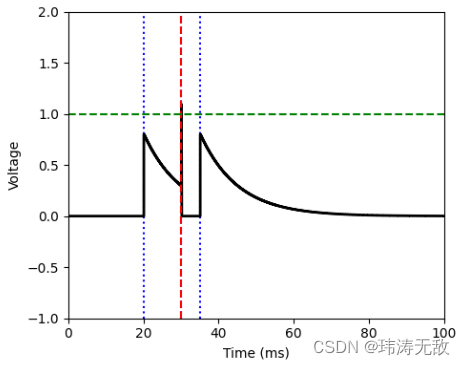``````import numpy as np
import matplotlib.pyplot as plt

fig = plt.figure(figsize=(5, 4))
ax = plt.subplot(111)

# Function that runs the simulation
# tau: time constant (in ms)
# t0, t1, t2: time of three input spikes
# w: input synapse weight
# threshold: threshold value to produce a spike
# reset: reset value after a spike
def LIF2(tau=10, taut=20, t0=20, t1=30, t2=35, w=0.8, threshold=1.0, dthreshold=0.5, reset=0.0):
# Spike times, keep sorted because it's more efficient to pop the last value off the list
times = [t0, t1, t2]
times.sort(reverse=True)
# set some default parameters
duration = 100 # total time in ms
dt = 0.1 # timestep in ms
alpha = np.exp(-dt/tau) # this is the factor by which V decays each time step
beta = np.exp(-dt/taut) # this is the factor by which Vt decays each time step
V_rec = [] # list to record membrane potentials
Vt_rec = [] # list to record threshold values
V = 0.0 # initial membrane potential
Vt = threshold
T = np.arange(np.round(duration/dt))*dt # array of times
spikes = [] # list to store spike times
# clear the axis and plot the spike times
ax.clear()
for t in times:
ax.axvline(t, ls=':', c='b')
# run the simulation
for t in T:
V_rec.append(V) # record
Vt_rec.append(Vt)
V *= alpha # integrate equations
Vt = (Vt-threshold)*beta+threshold
if times and t>times[-1]: # if there has been an input spike
V += w
times.pop() # remove that spike from list
V_rec.append(V) # record V before the reset so we can see the spike
Vt_rec.append(Vt)
if V>Vt: # if there should be an output spike
V = reset
Vt += dthreshold
spikes.append(t)
# plot everything (T is repeated because we record V twice per loop)
ax.plot(np.repeat(T, 2), V_rec, '-k', lw=2)
ax.plot(np.repeat(T, 2), Vt_rec, '--g', lw=2)
for t in spikes:
ax.axvline(t, ls='--', c='r')
ax.set_xlim(0, duration)
ax.set_ylim(-1, 2)
ax.set_xlabel('Time (ms)')
ax.set_ylabel('Voltage')
plt.tight_layout()
plt.show()
#display(fig)

LIF2()``````

运行结果为：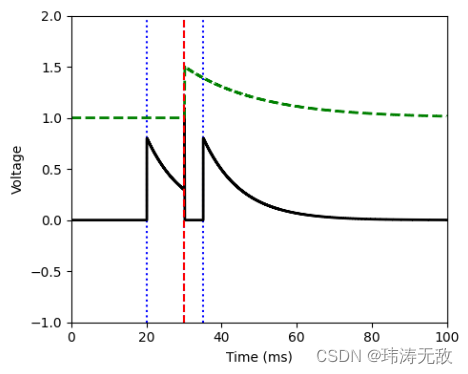# 二、脉冲神经网络前向传播过程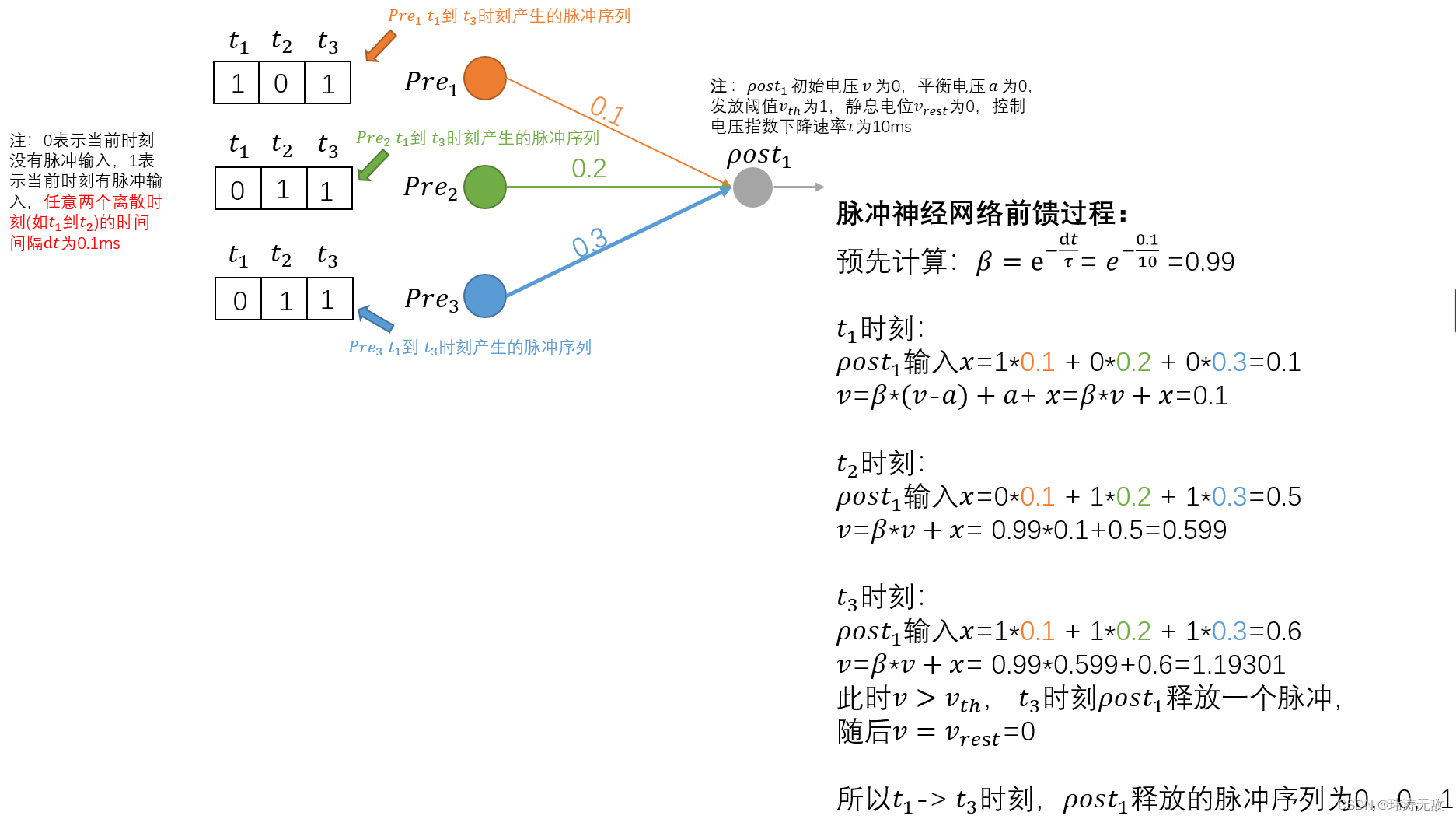# 三、脉冲神经网络突触权重学习方法(STDP)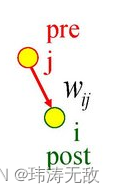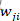的更新在脉冲神经网络中最常用的方法是STDP方法，STDP更新突触权重的方式是：若突触前脉冲比突触后脉冲到达时间早，会导致Long-Term Potentiation(LTP)效应，即权重会增加。反之，若突触前脉冲比突触后脉冲到达时间晚，会引起LTD，即权重会减小。在神经科学实验中，人们多次发现和验证了STDP是大脑突触权重更新的方式，突触权重更新意味着学习和信息的存储，也意味着大脑发育过程中神经元回路的发展和完善。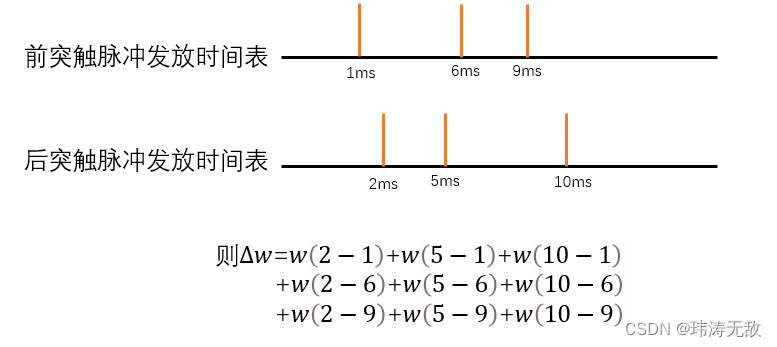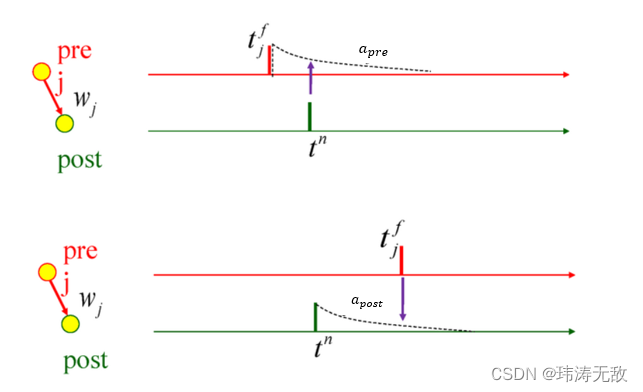痕迹变化由LIF模型控制：同理当突触后脉冲发放时：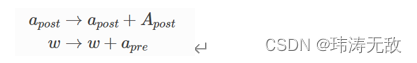# 四、泊松脉冲编码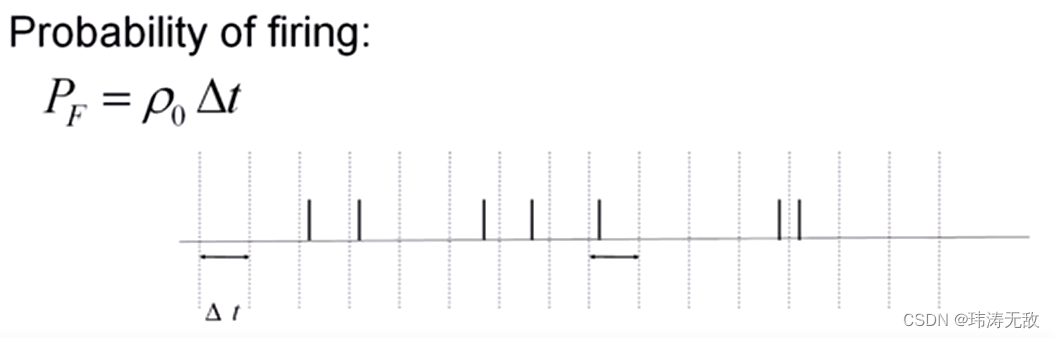# 最后，推荐一个可以用来学习脉冲神经网络特性的编程工具包：brian2

python安装方式：pip install brian2

(0)